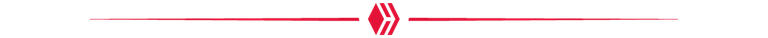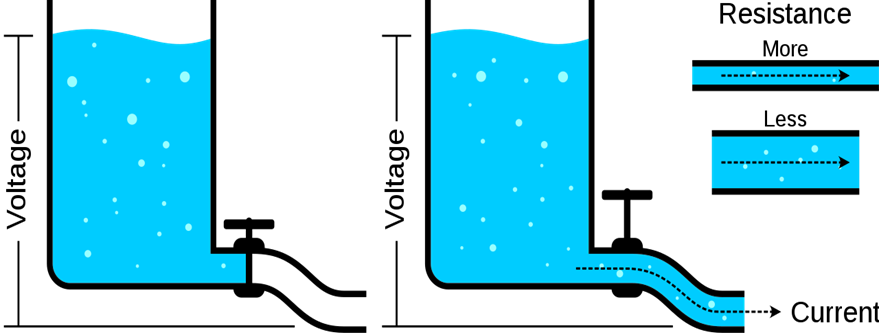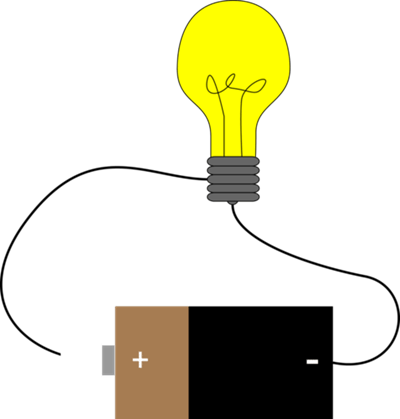# [Basics] The Difference Between Voltage and Current

in STEMGeeks7 months ago

# Back to Basics

Since every device requires an electrical power supply for its operation, it is necessary to understand the magnitudes that make possible the behavior of the electrical supply that provides energy to our refrigerators, microwaves and our homes, taking into account the main ones: Voltage and Current.

Although they are two different things, by the fact of being related when talking about any circuit many people tend to confuse them, talking about "the voltage flowing through" or "the current in", both phrases being erroneous approaches. In order to give more clarity to what the mystery of the voltage, also known as electric potential or tension; and the current or intensity represents, we will give a clear and concise explanation of what these two phenomena represent, at the same time showing how they act to generate "electricity".All credits to xegxef and Pixabay.com## VOLTAGE AND CURRENT: THE WATER TANK ANALOGY.

In technical language, voltage, tension or potential difference is the amount of potential energy existing between two points while current represents the amount of electrons flowing through a surface or element at a given time.Source

According to this illustration, initially we have a water tank with a closed pipe, which prevents the flow of water through it. Observing the amount of water, we know that gravity is constantly trying to push it down, where the pipe is, which it cannot do by the action of the faucet, so it can be identified as "potential". So, if we were to measure the difference between the potential of the amount of water in the tank and the amount of water at the end of the pipe (nothing), we would have the equivalent of our voltage.

If we were to open the faucet, the water in the tank would begin to flow through the pipe to its destination, having a "flow" of liquid through this point, being the equivalent of the current in this example.

Now, the flow of this water current can be controlled, making it greater or lesser according to a simple change: The thickness of the pipe: If we have that the pipe has a larger diameter, more water will pass through it in a specific time and therefore, it will be said that there is more "current", if the diameter is reduced, you will have a lower current. It is the opposition to the passage of water generated by the change in the thickness of the pipe that will be known as resistance.## In Electrical Terms

Now, if we want to understand how voltage and current work in electrical terms, we only need to change the water current by an electric current, the potential difference between two points of the tank by the difference between two terminals of a battery (voltage) and the force of gravity by the electromagnetic force as shown in the following network:Source

If we take a 9V battery and we connect it to a light bulb, closing this circuit by its ends, we will have that the light bulb will light up. At first we have to understand that according to the electromagnetic force, electrons in opposite charges attract and in equal charges repel. Thus, the electrons of the battery in the negative pole will be attracted by the positive pole of this same one, having that this flow of electrons will be our electrical current.

Now, if we had 0V no matter how high the current was, the electrons could not move, this because they lack the potential energy that makes them flow through the circuit.

In this way we can also clarify the misinterpretation coming from the phrase "The current kills", since although it is the flow of electrons through our body in the form of current that can harm us, what makes that in a principle the electrons can enter when entering in contact with us is the voltage.

In the case of our example, because the battery is 9V after being measured the potential difference between the positive and negative terminal of this, the electrons can pass without any problem by the network.

In addition to this, when passing through the bulb, the current generated will be limited due to the resistance of the bulb, causing the voltage to remain the same plus the current to be reduced in this load.## A Quick Glimpse to Ohm’s Law

If we want to explain this in mathematical terms, it suffices to use Ohm's law, postulated by the German physicist and mathematician Georg Simon Ohm, which dictates that voltage is simply the result of the product of current and resistance.

V=R*I (1)

In this way, to obtain resistance or current values, it is enough to solve for the unknown variables. For example, if we take again a 9V battery and assume that the resistance of the bulb is 10Ω, we would have that the current passing through it would be 0.9A.

Whether working with low power circuits or larger networks it is always necessary to have the necessary equipment and knowledge to measure and work with voltage and current, so the most important basis for undertaking projects related to electricity is the knowledge of these two magnitudes and the difference between them. Much success in your projects and good luck!Translated by DeepL

### Pages used for research

https://electronicsclub.info/voltage.htm#:~:text=Voltage%20is%20sometimes%20described%20as,current%20cannot%20flow%20without%20voltage.&text=current%20can%20flow.&text=current%20cannot%20flow.